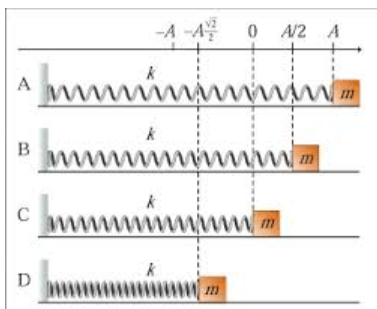# Problem: Figure 1 shows a harmonic oscillator at four different moments, labeled A, B, C, and D. Assume that the force constant k, the mass of the block, m, and the amplitude of vibrations, A, are given. We will also assume that there are no resistive forces so the total energy of the oscillator remains constant. Which moment corresponds to the maximum kinetic energy of the system? You can indicate the answer by the letter A, B, C, or D.

###### FREE Expert Solution

The kinetic energy is maximum at the equilibrium position. At the equilibrium position, displacement is zero.

81% (414 ratings)###### Problem Details

Figure 1 shows a harmonic oscillator at four different moments, labeled A, B, C, and D.Assume that the force constant k, the mass of the block, m, and the amplitude of vibrations, A, are given. We will also assume that there are no resistive forces so the total energy of the oscillator remains constant.

Which moment corresponds to the maximum kinetic energy of the system? You can indicate the answer by the letter A, B, C, or D.